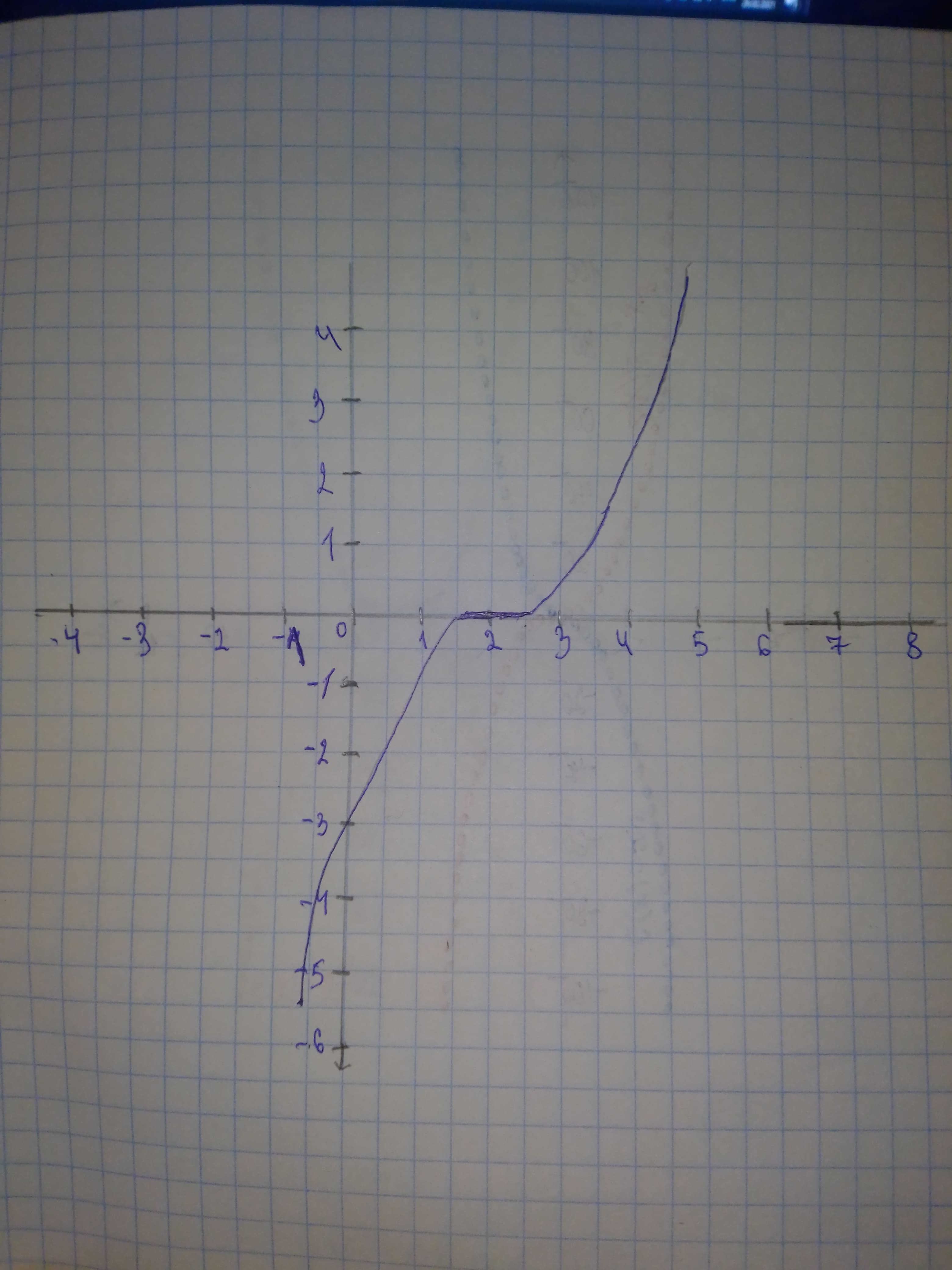# g is related to one of the six parent functions. (a) Identify the parent function f. (b) Describe the sequence of transformations from f to g. (c) Sketch the graph of g by hand. (d) Use function notation to write g in terms of the parent function f.g(x)=frac{1}{3}(x-2)^{3}usagirl007A 2021-02-06 Answered
g is related to one of the six parent functions. (a) Identify the parent function f. (b) Describe the sequence of transformations from f to g. (c) Sketch the graph of g by hand. (d) Use function notation to write g in terms of the parent function f.$g\left(x\right)=\frac{1}{3}{\left(x-2\right)}^{3}$
You can still ask an expert for help

• Questions are typically answered in as fast as 30 minutes

Solve your problem for the price of one coffee

• Math expert for every subject
• Pay only if we can solve itAGRFTr

a) Parent function: $f\left(x\right)={x}^{3}$ b) Horizontal shift 2 units to the right Vertical shrink by a factor of $\frac{1}{3}$ c) The graph will be:d) In function notation, Answer: $g\left(x\right)=\frac{1}{3}f\left(x-2\right)$